## Reflections: Geometry

Greetings and welcome to Mathsux! Today we are going to go over reflections geometry, one of the many types of transformations that come up in geometry. And thankfully, reflection transformation is one of the easiest types to master, especially if you’re more of a visual learner/artistic type person. In this post, we will go over a reflection across the line x=-2, but if you want to see a reflection across the x axis, the y axis, and the line y=x, please check out the math video below! So let’s get to it! And if you’re new to transformations, check out translations here.

## What are Reflections?

A Reflection on a coordinate plane is exactly what you think! A reflection is a type of transformation in geometry where we reflect a point, a line segment, or a shape over a line to create a mirror image of itself.  Think of the wings of a butterfly, a page being folded in half, or anywhere else where there is perfect symmetry, each of these are great examples of a reflection!

## Reflection Rules:

If you are completely new to reflections, you may want to check out the video above that goes into deriving reflection rules over the different axis. But for those of you more comfortable with reflection, check out the example below where we reflect the image of a triangle onto itself over the line x=-2.

## Example:

Step 1: First, let’s draw in line x=-2. Note that whenever we have x equal to a number, we end up drawing a vertical line at that point on the x axis, in this case at x=-2.

Step 2: Find the distance each point is from the line x=-2 and reflect it on the other side, measuring the same distance.

First, let’s look at point C, notice it’s 1 unit away from the line x=-2 on the right. To reflect point c, we are going to count 1 unit but this time to the left of the line x=-2 and label our new point, C|.

Step 3: Now we are going to reflect coordinate point A in much the same way! Notice that point A is 2 units away on the left of line x=-2, we then want to measure 2 units to the right of our line x=-2 and mark our new coordinate point, A|.

Step 4: Lastly, we want to reflect coordinate point B. This time, point B is 1 unit away on the right side of the line x=-2, we then measure 1 unit to the opposite direction of our line, x=-2, and mark our new point, B|.

Step 5: Now that we have all the newly reflected coordinate points of our triangle, finally, we can now connect them all, for our fully reflected image of right triangle A|B|C|.

Notice our newly reflected triangle is not just a mirror image of itself, but when the original figure is reflected it actually ends up overlapping onto itself!? How did this happen? That is because this our reflection line came right down the middle of our original image, triangle ABC. Shapes that reflect onto themselves are a bit tricky but not impossible, just remember to measure out the distance of each coordinate point and reflections should be a breeze!

## Rigid Motion:

Reflections are a special type of transformation in geometry that maintains rigid motion, meaning when a point, line, or shape is reflected the angles, and line segments retain their value. For example, if we were to measure the area of both right triangles, before and after reflection, we would find the areas to remain unchanged. Meaning the area of triangle ABC is equal to the area of triangle A|B|C| . Another rigid transformation includes rotations and translations.

Looking to practice your new reflection skills? Try the reflection practice problems below, with solutions to each question, to truly master the topic! Happy calculating!

## Solutions:

Still got questions?  No problem! Check out the video above or comment below! There is also a bonus video if you scroll all the way down at the end of this post for anyone who wants to see how to reflect a line over the line x=2. See how it differs and how it is similar to the example shown here. Happy calculating! 🙂

Don’t forget to follow MathSux on social media for the latest tips and tricks to ace math!

And if you are looking for more Transformations Check out the related posts below and let me know if you have any questions? Or maybe you just want to share your favorite type of transformation? Either way, happy calculating!

Translations

Dilations

Rotation

## Piecewise Functions: Algebra

Greetings, today’s post is for those in need of a piecewise functions review!  This will cover how to graph each part of that oh so intimidating piecewise functions.  There’s x’s, there are commas, there are inequalities, oh my! We’ll figure out what’s going on here and graph each part of the piecewise-function one step at a time.  Then check yourself with the practice questions at the end of this post. Happy calculating! 🙂

## What are Piece-Wise Functions?

Exactly what they sound like! A function that has multiple pieces or parts of a function.  Notice our function below has different pieces/parts to it.  There are different lines within, each with their own domain.

Now let’s look again at how to solve our example, solving step by step:

Translation: We are going to graph the line f(x)=x+1 for the domain where x > 0

To make sure all our x-values are greater than or equal to zero, we create a table plugging in x-values greater than or equal to zero into the first part of our function, x+1.  Then plot the coordinate points x and y on our graph.

Translation: We are going to graph the line  f(x)=x-3 for the domain where x < 0.

To make sure all our x-values are less than zero, let’s create a table plugging in negative x-values values leading up to zero into the second part of our function, x-3.  Then plot the coordinate points x and y on our graph.

## Practice Questions:

Graph each piecewise function:

## Solutions:

Still got questions?  No problem! Check out the video above or comment below for any questions. Happy calculating! 🙂

***Bonus! Want to test yourself with a similar NYS Regents question on piecewise functions?  Click here.

## Intersecting Secants Theorem: Geometry

Ahoy! Today we’re going to cover the Intersecting Secants Theorem!  If you forgot what a secant is in the first place, don’t worry because all it is a line that goes through a circle.  Not so scary right? I was never scared of lines that go through circles before, no reason to start now.

If you have any questions about anything here, don’t hesitate to comment below and check out my video for more of an explanation. Stay positive math peeps and happy calculating! 🙂

Wait, what are Secants?

Intersecting Secants Theorem: When secants intersect an amazing thing happens! Their line segments are in proportion, meaning we can use something called the Intersecting Secants Theorem to find missing line segments.  Check it out below:

Let’s now see how we can apply the intersecting Secants Theorem to find missing length.

Step 1: First, let’s write our formula for Intersecting Secants.

Step 2: Now fill in our formulas with the given values and simplify.

Step 3: All we have to do now is solve for x! I use the product.sum method here, but choose the factoring method that best works for you!

Step 4: Since we have to reject one of our answers, that leaves us with our one and only solution x=2.

Practice Questions: Find the value of the missing line segments x.

Solutions:

Still got questions?  No problem! Check out the video above or comment below for any questions and follow for the latest MathSux posts. Happy calculating! 🙂

To review a similar NYS Regents question check out this post here.

## Circle Theorems & Formulas

Inscribed Angles & Intercepted Arcs

Area of a Sector

Circle Theorems

## Absolute Value Equations: Algebra

Happy Wednesday math friends! Today, we’re going to go over how to solve absolute value equations.  Solving for absolute value equations supplies us with the magic of two potential answers since absolute value is measured by the distance from zero.  And if this sounds confusing, fear not, because everything is explained below!

Also, if you have any questions about anything here, don’t hesitate to comment. Happy calculating! 🙂

Absolute Value measures the “absolute value” or absolute distance from zero.  For example, the absolute value of 4 is 4 and the absolute value of -4 is also 4.  Take a look at the number line below for a clearer picture: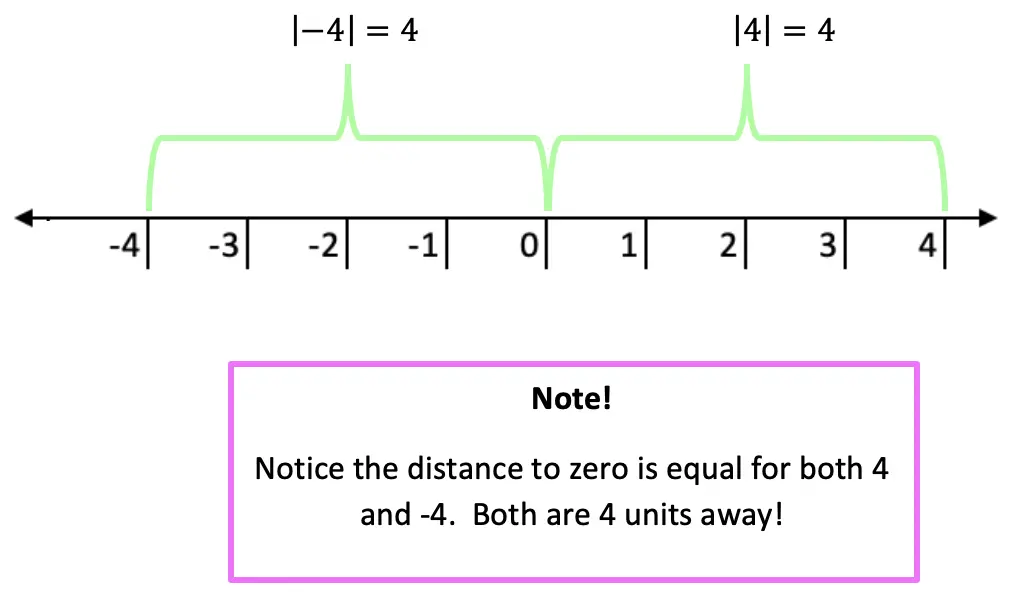Now let’s see how we can apply our knowledge of absolute value equations when there is a missing variable!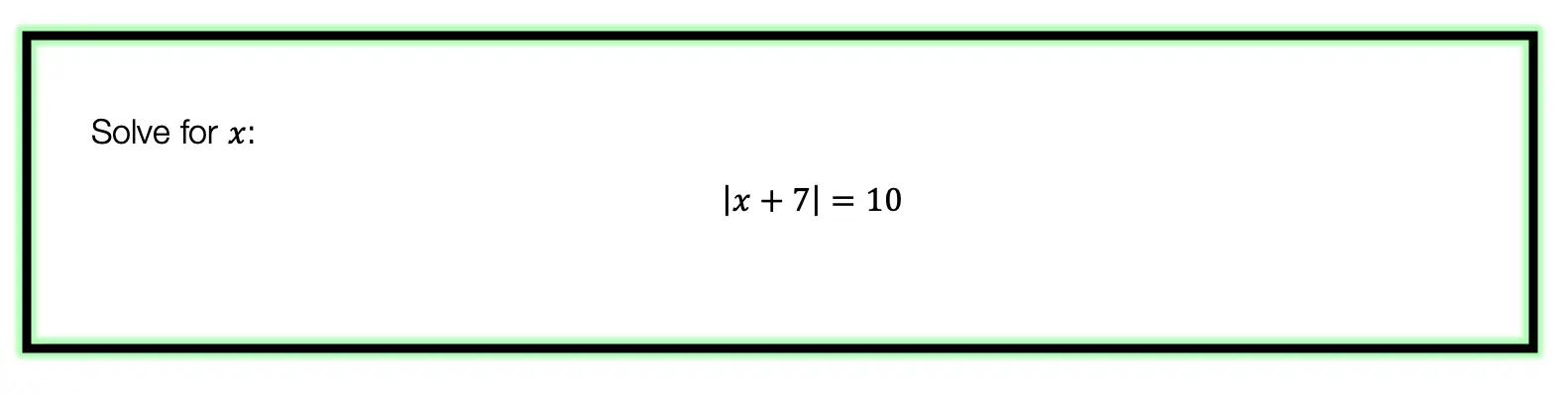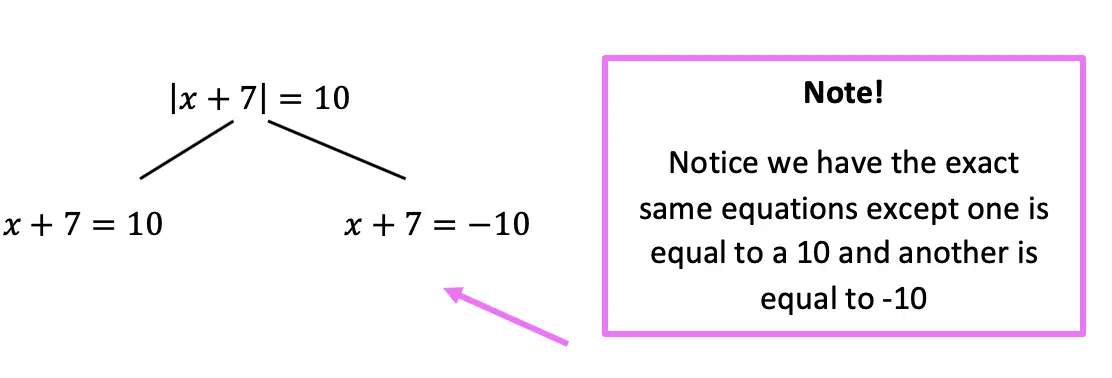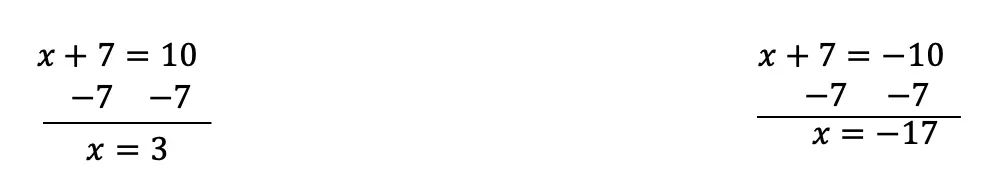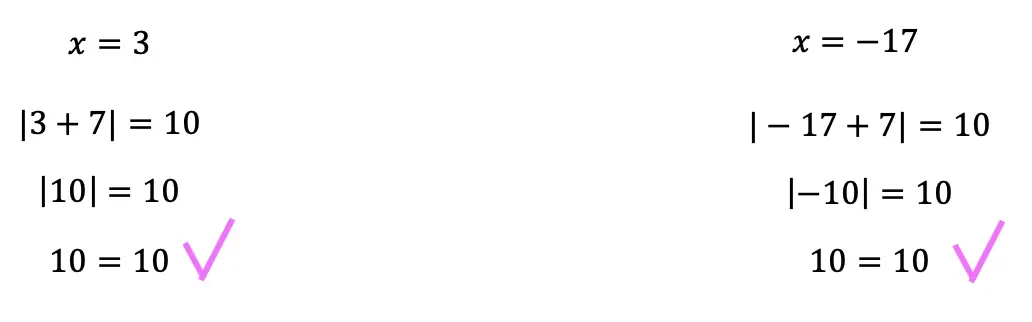Now let’s look at a slightly different example: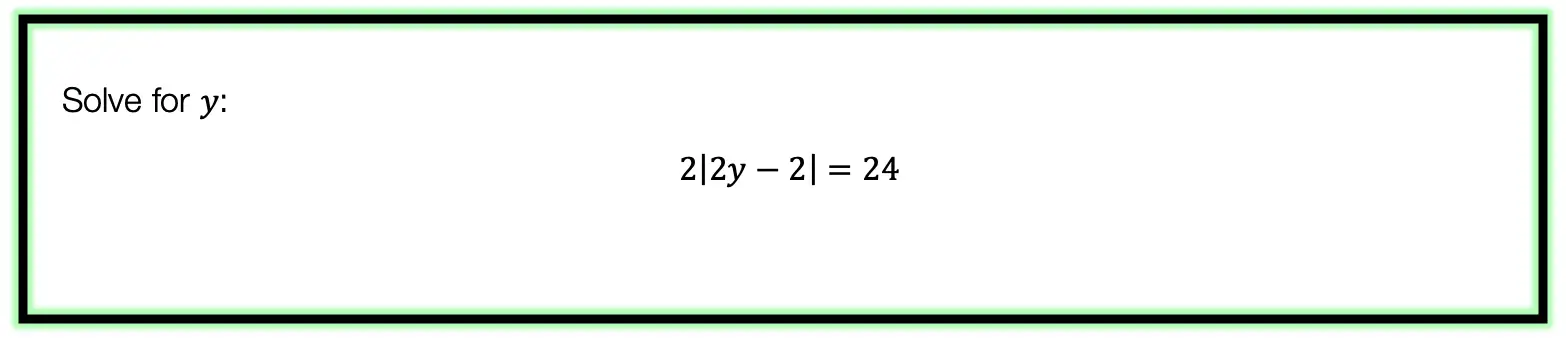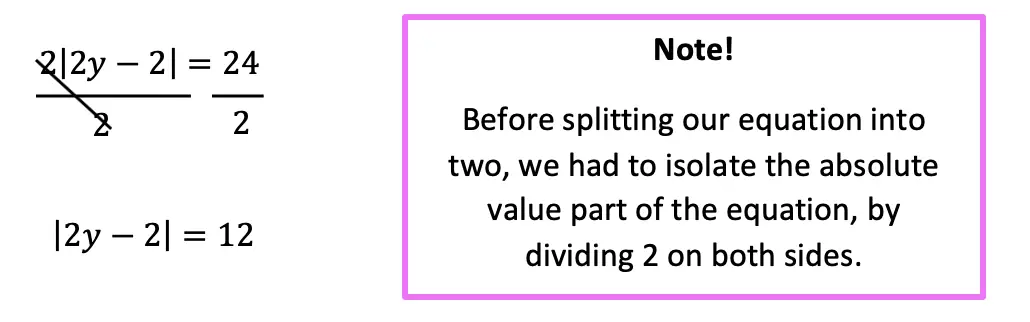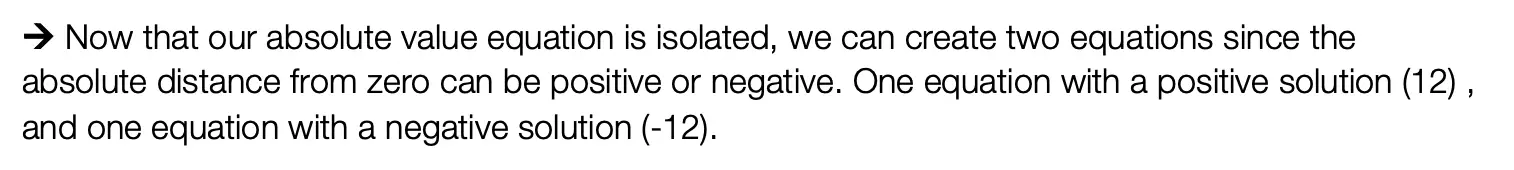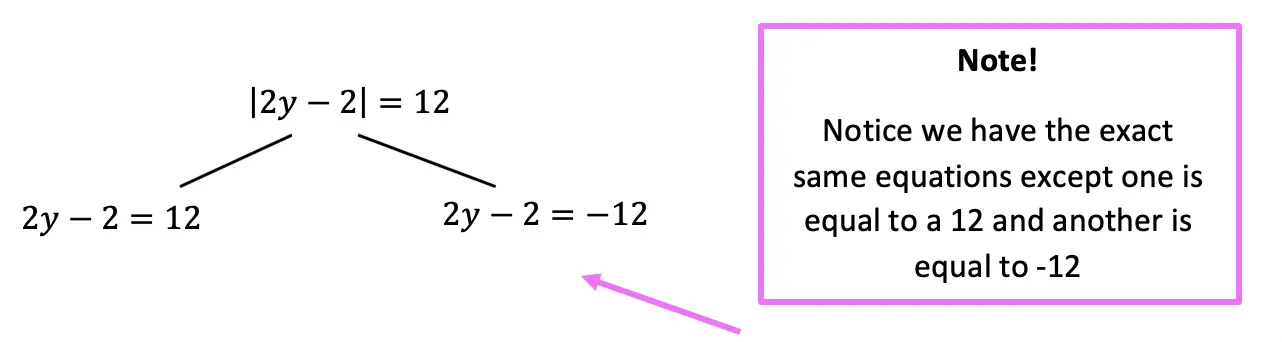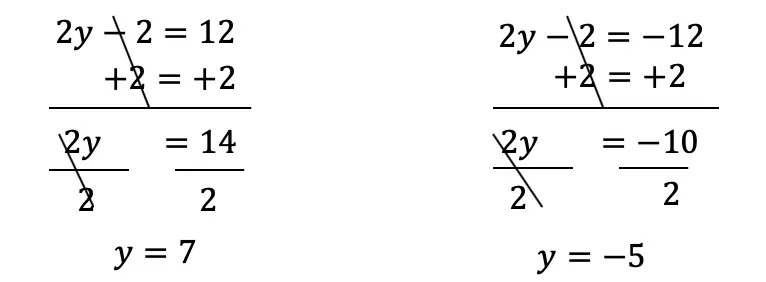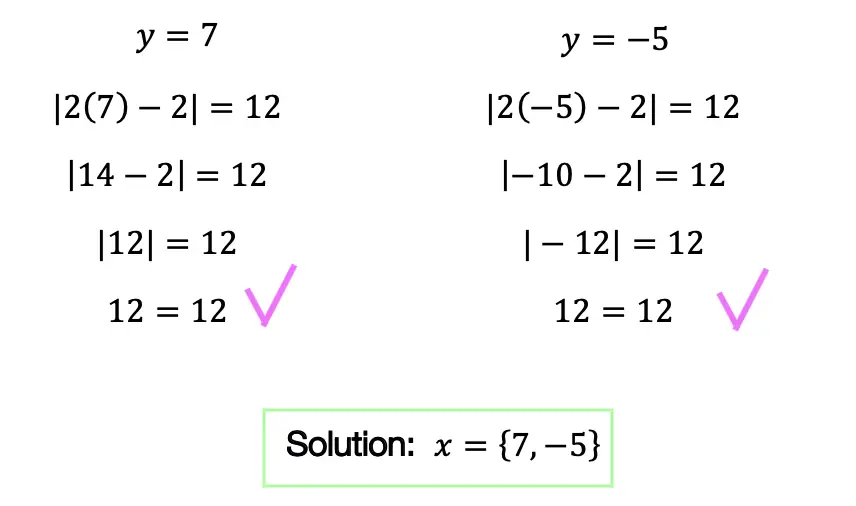Practice Questions: Given the following right triangles, find the missing lengths and side angles rounding to the nearest whole number.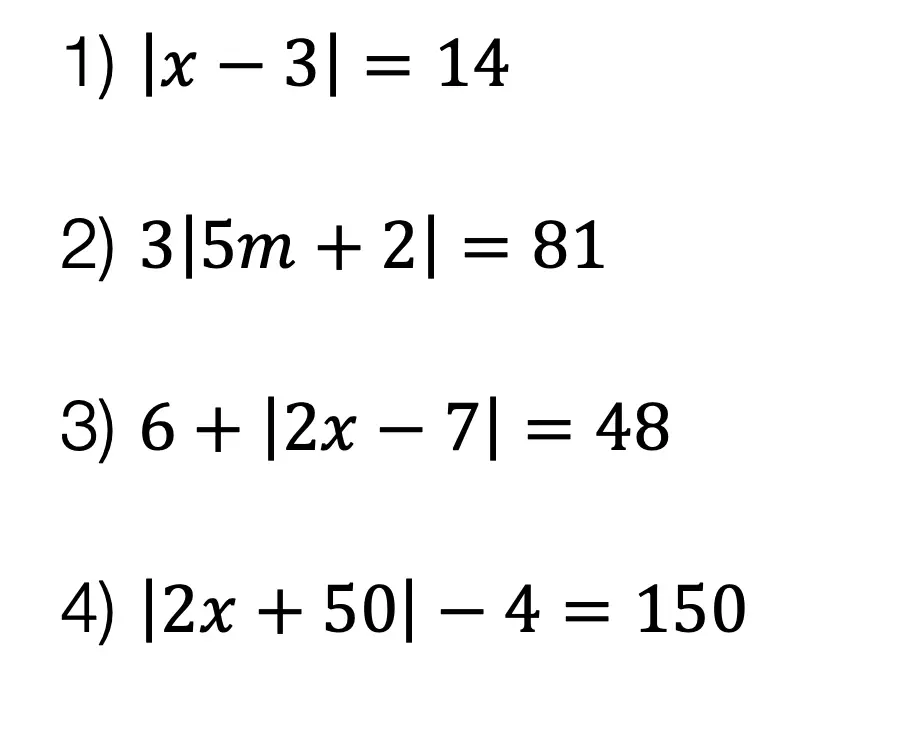Solutions: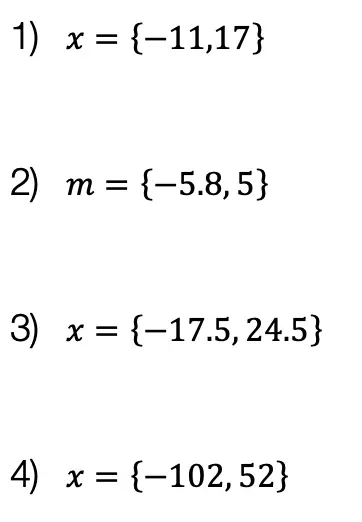Still got questions?  No problem! Check out the video the same examples outlined above. Happy calculating! 🙂

Also, if you’re looking for a review on combining like terms and the distributive property, check out this post here.

## How to use SOH CAH TOA: Geometry

Greeting math friends and welcome to Mathsux! In this post, we are going to start with the very basics of trigonometry by going over how to find a missing angle and/or side length of right triangles while using the famous trigonometric function sine, cosine, or tangent, (aka how to use SOH CAH TOA).  Woo hoo! These are the basics of right triangle trigonometry, and provide the base for mastering so many more interesting things to come in trigonometry! So, let’s get to it!

SOH CAH TOA is an acronym that stands for the following trig functions and parts of a right triangle. We’ll explain more in this post!

SOH CAH TOA

Also, if you have any questions about anything here, don’t hesitate to comment below or watch the video below. Also, don’t forget to subscribe to MathSux for FREE math videos, lessons, and practice questions every week. Happy calculating! 🙂

## What does SOH CAH TOA stand for?

A Trigonometric Ratio, more commonly known as Sine, Cosine, and Tangent, are trig ratios that naturally exist within a right triangle.  This means that the sides and angles of a right triangle are in proportion within itself.  It also means that if we are missing a side or an angle of a right triangle, based on what we’re given, we can figure out what the value of the sides or angles are, based on these ratios!

Let’s take a look at what Sine, Cosine, and Tangent are all about!

Ready for your first right angled triangle example? Check it out below!

## SOH CAH TOA Example #1:

Now let’s see how we can apply trig ratios when there is a missing side or angle in a right triangle!

Step 1: First, let’s identify the different sides of our right triangle depending on which angle we are focusing on, which in this case is a 60º angle. Based on the locations of our angle, we can label each side as the hypotenuse, adjacent, or the opposite.

Notice below, that the opposite side labeled x, is labeled the “opposite” side because it is opposite to our given angle, 60º. The remaining side is considered the adjacent side of our triangle because it is directly next to our given angle, 60º.

Step 2: Now, let’s write out SOH CAH TOA. Notice the only trig function that uses both the hypotenuse and the opposite is sine! Knowing to use the sine function, let’s fill in our formula using the hypotenuse = 5 and opposite = x in order to find the value for missing side length x.

In order to use the sin function correctly, we’re going to need to plug in our given angle, which is 60º, and then set up our proportion. sin(60º)=x/5. By using the sine function, our calculator, and a little bit of algebra we’ll be able to solve for the unknown side.

Ready for another example?! Check out another SOH CAH TOA problem using right triangles below!

## Right Triangle Trig Example #2:

Step 1: First, let’s identify the different parts of the right triangle we are given (the hypotenuse, adjacent, and the opposite). Notice in this example, we are given the adjacent and hypotenuse and need to find the value of the unknown angle, θ.

Step 2: Next, let’s write out our acronym, SOH CAH TOA, to see which trig function can help us with our question! Notice the only trig function that uses both adjacent and hypotenuse is cosine. This is what we will use to solve for the unknown angle, θ.

We use cosine, by setting up our proportion, cos(θ)=adjacent/ hypotenuse, knowing we can then plug in 12 for our adjacent value, and 13 for our hypotenuse value.

Think you’re ready to test out SOH CAH TOA on your own? Try the following Practice Questions on your own!

## Practice Questions:

Given the following right triangles, find the missing lengths and side angles rounding to the nearest whole number.

## Solutions:

Still got questions?  No problem! Check out the video the same examples outlined above and happy calculating! 🙂

## Related Trigonometry Posts:

The Unit Circle

Basic Right Triangle Trigonometric Ratios (SOH CAH TOA)

4545 90 Special Triangles

30 60 90 Special Triangles

Graphing Trig Functions

Transforming Trig Functions

Factoring Trig Functions

Law of Cosines

Trig Identities

Law of Sines

## Perpendicular & Parallel Lines Through a Given Point: Geometry

Happy Wednesday math friends! Today we’re going to go over the difference between perpendicular and parallel lines, then we’ll use our knowledge of the equation of a line (y=mx+b) to see how to find perpendicular and parallel lines through a given point.  This is a common question that comes up on the NYS Geometry Regents and is something we should prepare for, so let’s go!

If you need any further explanation, don’t hesitate to check out the Youtube video below that goes into detail on how to find perpendicular and parallel lines through a given point one step at a time. Happy calculating! 🙂

## Perpendicular Lines:

Perpendicular Lines: Lines that intersect to create a 90-degree angle and can look something like the graph below.  Their slopes are negative reciprocals of each other which means they are flipped and negated. See below for example!

Example: Find an equation of a line that passes through the point (1,3) and is perpendicular to line y=2x+1 .

## Parallel Lines:

Parallel lines are lines that go in the same direction and have the same slope (but have different y-intercepts). Check out the example below!

Example: Find an equation of a line that goes through the point (-5,1) and is parallel to line y=4x+2.

Try the following practice questions on your own!

## Practice Questions:

1) Find an equation of a line that passes through the point (2,5) and is perpendicular to line y=2x+1.

2) Find an equation of a line that goes through the point (-2,4) and is perpendicular to line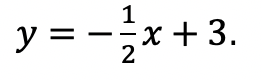3)  Find an equation of a line that goes through the point (1,6) and is parallel to line y=3x+2.

4)  Find an equation of a line that goes through the point (-2,-2)  and is parallel to line y=2x+1.

## Solutions:

Need more of an explanation? Check out the video that goes over these types of questions up on Youtube (video at top of post) and let me know if you have still any questions.

Happy Calculating! 🙂

Looking for more on Perpendicular and parallel lines? Check out this Regents question on perpendicular lines here!